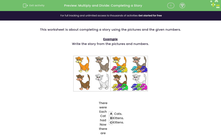# Complete a number story

In this worksheet, students complete a multiplication or division task from the given information.Key stage:  KS 2

Curriculum topic:   Number: Multiplication and Division

Curriculum subtopic:   Solve Multiplication/Division Problems

Difficulty level:#### Worksheet Overview

In this activity, we will be using pictures to complete a number story and calculation.

Look at the pictures below.We can see that on the left, there are 4 cats

On the right are the same 4 cats, but this time they each have 3 kittens.

There are A cats, each one has B kittens.

Altogether there are C kittens.

A = 4

B = 3

C = 12

To find the total number of kittens, we could use these numbers to write a calculation.

4 × 3 = 12

There are 12 kittens in total.

Let's try an example question together.

ExampleWrite a calculation to show how many cookies each child gets.

First, let's think about the numbers involved in this story.

There are A children, they bake B cookies.

C is the share they each get.

A = 2

B = 8

C = 4

So, there are 8 cookies to be shared between 2 children.

8 ÷ 2 = 4